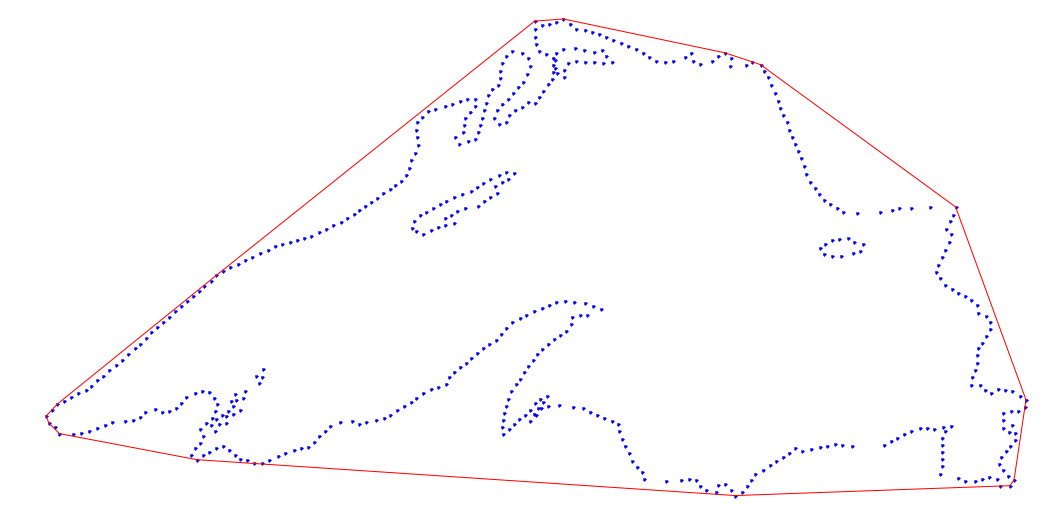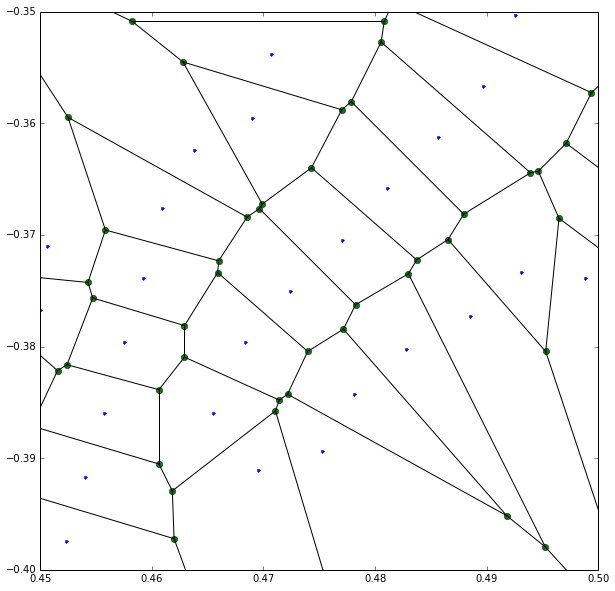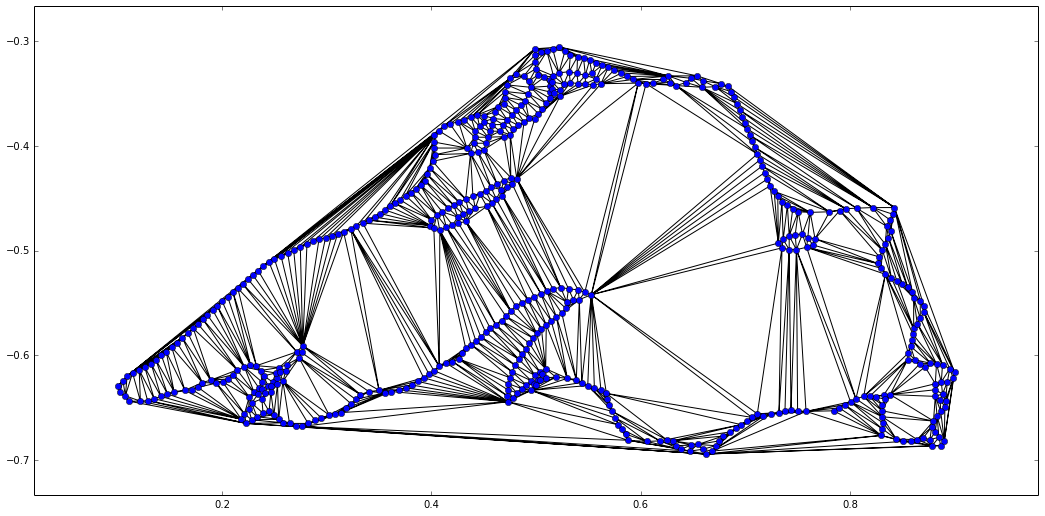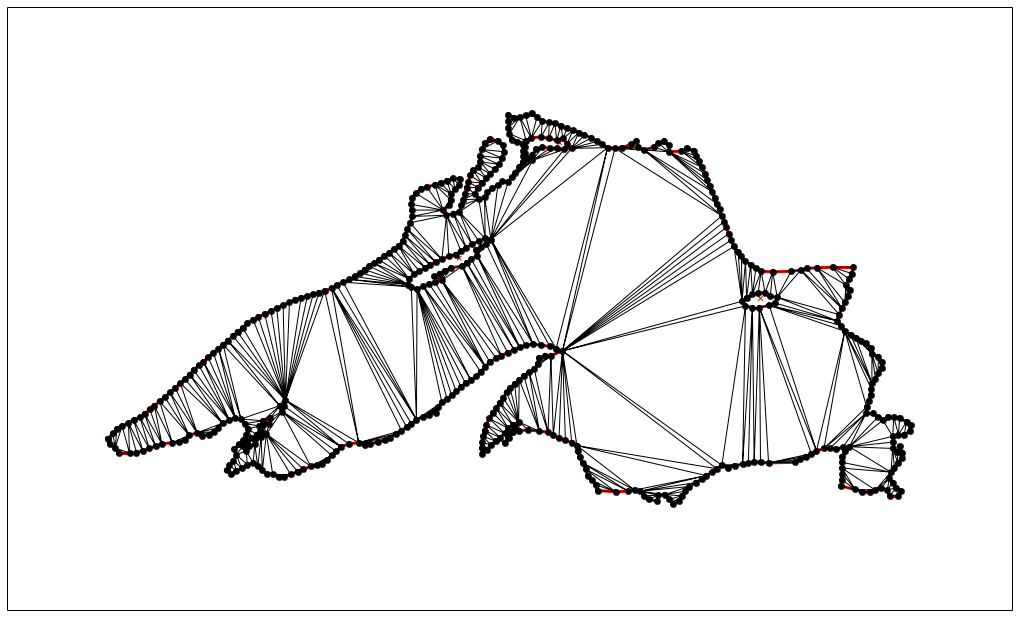# Computational Geometry in Python¶

Computational Geometry is a field of mathematics that seeks the development of efficient algorithms to solve problems described in terms of basic geometrical objects. We differentiate between Combinatorial Computational Geometry and Numerical Computational Geometry.

• Combinatorial Computational Geometry deals with interaction of basic geometrical objects: points, segments, lines, polygons, and polyhedra. In this setting we have three categories of problems:

• Static Problems: The construction of a known target object is required from a set of input geometric objects.

• Geometric Query Problems: Given a set of known objects (the search space) and a sought property (the query), these problems deal with the search of objects that satisfy the query.

• Dynamic Problems: Similar to problems from the previous two categories, with the added challenge that the input is not known in advance, and objects are inserted or deleted between queries/constructions.

• Numerical Computational Geometry deals mostly with representation of objects in space described by means of curves, surfaces, and regions in space bounded by those.

Before we proceed to the development and analysis of the different algorithms in those two settings, it pays off to explore the basic background: Plane Geometry.

## Plane Geometry¶

The basic Geometry capabilities are usually treated through the Geometry module of the sympy libraries. Rather than giving an academical description of all objects and properties in that module, we discover the most useful ones through a series of small self-explanatory python sessions.

### Points, Segments¶

We start with the concepts of point and segment. The aim is to illustrate how easily we can check for collinearity, compute lengths, midpoints, or slopes of segments, for example. We also show how to quickly compute the angle between two segments, as well as deciding whether a given point belongs to a segment or not. The next diagram illustrates an example, which we follow up with code.In :
from sympy.geometry import *

P1 = Point(0, 0)
P2 = Point(3, 4)
P3 = Point(2, -1)
P4 = Point(-1, 5)

S1 = Segment(P1, P2)
S2 = Segment(P3, P4)

In :
Point.is_collinear(P1, P2, P3)

Out:
False
In :
S1.length

Out:
5
In :
S2.midpoint

Out:
Point(1/2, 2)
In :
S1.slope

Out:
4/3
In :
S1.intersection(S2)

Out:
[Point(9/10, 6/5)]
In :
Segment.angle_between(S1, S2)

Out:
acos(-sqrt(5)/5)
In :
S1.contains(P3)

Out:
False

### Lines¶

The next logical geometrical concept is the line. We can perform more interesting operations with lines, and to that effect we have a few more constructors. We can find their equations, compute the distance between a point and a line, and many other operations.In :
L1 = Line(P1, P2)

L2 = L1.perpendicular_line(P3)  # perpendicular line to L1

In :
L2.arbitrary_point()            # parametric equation of L2

Out:
Point(4*t + 2, -3*t - 1)
In :
L2.equation()                   # algebraic equation of L2

Out:
3*x + 4*y - 2
In :
L2.contains(P4)                 # is P4 in L2?

Out:
False
In :
L2.distance(P4)                 # distance from P4 to L2

Out:
3
In :
L1.is_parallel(S2)              # is S2 parallel to L1?

Out:
False

### Circles¶

The next geometrical concept we are to explore is the circle. We may define a circle by its center and radius, or by three points on it. We can easily compute all of its properties.In :
C1 = Circle(P1, 3)
C2 = Circle(P2, P3, P4)

In :
C2.area

Out:
1105*pi/98
In :
C2.radius

Out:
sqrt(2210)/14
In :
C2.equation()

Out:
(x - 5/14)**2 + (y - 27/14)**2 - 1105/98
In :
C2.center

Out:
Point(5/14, 27/14)
In :
C2.circumference

Out:
sqrt(2210)*pi/7

Computing intersections with other objects, checking whether a line is tangent to a circle, or finding the tangent lines through an non-interior point, are simple tasks too:

In :
C2.intersection(C1)

Out:
[Point(55/754 + 27*sqrt(6665)/754, -5*sqrt(6665)/754 + 297/754),
Point(-27*sqrt(6665)/754 + 55/754, 297/754 + 5*sqrt(6665)/754)]
In :
C2.intersection(S1)

Out:
[Point(3, 4)]
In :
C2.is_tangent(L2)

Out:
False
In :
C1.tangent_lines(P4)

Out:
[Line(Point(-1, 5), Point(-9/26 + 15*sqrt(17)/26, 3*sqrt(17)/26 + 45/26)),
Line(Point(-1, 5), Point(-15*sqrt(17)/26 - 9/26, -3*sqrt(17)/26 + 45/26))]

### Triangles¶

One of the most useful basic geometric concept is the triangle. We need robust and fast algorithms to manipulate and extract information from them. Let us show first the definition of one, together with a series of queries to describe its properties:

In :
T = Triangle(P1, P2, P3)

In :
T.area                          # Note it gives a signed area

Out:
-11/2
In :
T.angles

Out:
{Point(0, 0): acos(2*sqrt(5)/25),
Point(2, -1): acos(3*sqrt(130)/130),
Point(3, 4): acos(23*sqrt(26)/130)}
In :
T.sides

Out:
[Segment(Point(0, 0), Point(3, 4)),
Segment(Point(2, -1), Point(3, 4)),
Segment(Point(0, 0), Point(2, -1))]
In :
T.perimeter

Out:
sqrt(5) + 5 + sqrt(26)
In :
T.is_right()                    # Is T a right triangle?

Out:
False
In :
T.is_equilateral()              # Is T equilateral?

Out:
False
In :
T.is_scalene()                  # Is T scalene?

Out:
True
In :
T.is_isosceles()                # Is T isosceles?

Out:
False

Next, note how easily we can obtain representation of the different segments, centers, and circles associated with triangles, as well as the medial triangle (the triangle with vertices at the midpoints of the segments).

In :
T.altitudes

Out:
{Point(0, 0): Segment(Point(0, 0), Point(55/26, -11/26)),
Point(2, -1): Segment(Point(6/25, 8/25), Point(2, -1)),
Point(3, 4): Segment(Point(4/5, -2/5), Point(3, 4))}
In :
T.orthocenter                  # Intersection of the altitudes

Out:
Point(10/11, -2/11)
In :
T.bisectors()                  # Angle bisectors

Out:
{Point(0, 0): Segment(Point(0, 0), Point(sqrt(5)/4 + 7/4, -9/4 + 5*sqrt(5)/4)),
Point(2, -1): Segment(Point(3*sqrt(5)/(sqrt(5) + sqrt(26)), 4*sqrt(5)/(sqrt(5) + sqrt(26))), Point(2, -1)),
Point(3, 4): Segment(Point(-50 + 10*sqrt(26), -5*sqrt(26) + 25), Point(3, 4))}
In :
T.incenter                     # Intersection of angle bisectors

Out:
Point((3*sqrt(5) + 10)/(sqrt(5) + 5 + sqrt(26)), (-5 + 4*sqrt(5))/(sqrt(5) + 5 + sqrt(26)))
In :
T.incircle

Out:
Circle(Point((3*sqrt(5) + 10)/(sqrt(5) + 5 + sqrt(26)), (-5 + 4*sqrt(5))/(sqrt(5) + 5 + sqrt(26))), -11/(sqrt(5) + 5 + sqrt(26)))
In :
T.inradius

Out:
-11/(sqrt(5) + 5 + sqrt(26))
In :
T.medians

Out:
{Point(0, 0): Segment(Point(0, 0), Point(5/2, 3/2)),
Point(2, -1): Segment(Point(3/2, 2), Point(2, -1)),
Point(3, 4): Segment(Point(1, -1/2), Point(3, 4))}
In :
T.centroid                   # Intersection of the medians

Out:
Point(5/3, 1)
In :
T.circumcenter               # Intersection of perpendicular bisectors

Out:
Point(45/22, 35/22)
In :
T.circumcircle

Out:
Circle(Point(45/22, 35/22), 5*sqrt(130)/22)
In :
T.circumradius

Out:
5*sqrt(130)/22
In :
T.medial

Out:
Triangle(Point(3/2, 2), Point(5/2, 3/2), Point(1, -1/2))

Some other interesting operations with triangles:

• Intersection with other objects
• Computation of the minimum distance from a point to each of the segments.
• Checking whether two triangles are similar.
In :
T.intersection(C1)

Out:
[Point(9/5, 12/5), Point(sqrt(113)/26 + 55/26, -11/26 + 5*sqrt(113)/26)]
In :
T.distance(T.circumcenter)

Out:
sqrt(26)/11
In :
T.is_similar(Triangle(P1, P2, P4))

Out:
False

The other basic geometrical objects currently coded in the Geometry module are

• LinearEntity. This is a superclass (which we never use directly), with three subclasses Segment, Line and Ray. LinearEntity enjoys the following basic methods:

• are_concurrent(o1, o2, ..., on)
• are_parallel(o1, o2)
• are_perpendicular(o1, o2)
• parallel_line(self, Point)
• perpendicular_line(self, Point)
• perpendicular_segment(self, Point)
• Ellipse. An object with a center, together with horizontal and vertical radii. Circle is, as a matter of fact, a subclass of Ellipse with both radii equal.

• Polygon. A superclass we can instantiate by listing a set of vertices. Triangles are a subclass of Polygon, for example. The basic methods of Polygon are

• area
• perimeter
• centroid
• sides
• vertices
• RegularPolygon. This is a subclass of Polygon, with extra attributes:

• apothem
• center
• circumcircle
• exterior_angle
• incircle
• interior_angle
• radius

For more information about this module, refer to the official sympy documentation at docs.sympy.org/dev/modules/geometry.html

### Curves¶

There is also a non-basic geometric object: A Curve, which we define by providing parametric equations, together with the interval of definition of the parameter. It currently does not have many useful methods, other than those describing its constructors. Let us illustrate how to deal with these objects. For example, a three-quarters arc of an ellipse could be coded as follows:

In :
from sympy import var, pi, sin, cos

var('t', real=True)

Arc = Curve((3*cos(t), 4*sin(t)), (t, 0, 3*pi/4))


### Affine Transformations¶

To end the exposition on basic objects from the geometry module in the sympy library, we must mention that we may apply any basic affine transformations to any of the previous objects. This is done by combination of the methods reflect, rotate, translate and scale.

In :
T.reflect(L1)

Out:
Triangle(Point(0, 0), Point(3, 4), Point(-38/25, 41/25))
In :
T.rotate(pi/2, P2)

Out:
Triangle(Point(7, 1), Point(3, 4), Point(8, 3))
In :
T.translate(5,4)

Out:
Triangle(Point(5, 4), Point(8, 8), Point(7, 3))
In :
T.scale(9)

Out:
Triangle(Point(0, 0), Point(27, 4), Point(18, -1))
In :
Arc.rotate(pi/2, P3).translate(pi,pi).scale(0.5)

Out:
Curve((-2.0*sin(t) + 0.5 + 0.5*pi, 3*cos(t) - 3 + pi), (t, 0, 3*pi/4))

With these basic definitions and operations, we are ready to address more complex situations. Let us explore these new challenges next.

## Combinatorial Computational Geometry¶

Also called algorithmic geometry, the applications of this field are plenty: in robotics, these are used to solve visibility problems, and motion planning, for instance. Similar applications are employed to design route planning or search algorithms in geographic information systems (GIS).

Let us describe the different categories of problems, making emphasis on the tools for solving them that are available in the scipy stack.

### Static Problems¶

The fundamental problems in this category are the following:

• Convex hulls: Given a set of points in space, find the smallest convex polyhedron containing them.
• Voronoi diagrams: Given a set of points in space (the seeds), compute a partition in regions consisting of all points closer to each seed.
• Triangulations: Partition the plane with triangles, in a way that two triangles are either disjoint, or otherwise they share an edge or a vertex. There are different triangulations depending on the input objects, or constraints on the properties of the triangles.
• Shortest paths: Given a set of obstacles in a space, and two points, find the shortest path between the points that does not intersect any of the obstacles.

The problems of computation of convex hulls, basic triangulations, and Voronoi diagrams are intimately linked. The theory that explains this beautiful topic is explained in detail in a monograph in Computer Science titled Computational Geometry, written by Franco Preparata and Michael Shamos. It was published by Springer-Verlag in 1985.

#### Convex Hulls¶

While it is possible to compute the convex hull of a reasonably large set of points in the plane through the geometry module of the library sympy, this is not recommendable. A much faster and reliable code is available in the module scipy.spatial through the routine ConvexHull, which is in turn a wrapper to qconvex, from the Qhull libraries www.qhull.org. This routine also allows the computation of convex hulls in higher dimensions. Let us compare both methods, with the famous Lake Superior polygon, superior.poly.

poly files represent planar straight line graphs---a simple list of vertices and edges, together with information about holes and concavities, in some cases. The running example can be downloaded from www.math.sc.edu/~blanco/superior.poly. It contains a polygonal description of the coastline of Lake Superior, with 7 holes (for the islands), 518 vertices, and 518 edges.

For a complete description of the poly format, refer to www.cs.cmu.edu/~quake/triangle.poly.html. With that information, we can write a simple reader without much effort. This is an example:

In :
from numpy import array

"""
Simple poly-file reader, that creates a python dictionary
with information about vertices, edges and holes.
It assumes that vertices have no attributes or boundary markers.
It assumes that edges have no boundary markers.
No regional attributes or area constraints are parsed.
"""

output = {'vertices': None, 'holes': None, 'segments': None}

# open file and store lines in a list
file = open(file_name, 'r')
file.close()
lines = [x.strip('\n').split() for x in lines]

# Store vertices
vertices= []
N_vertices, dimension, attr, bdry_markers = [int(x) for x in lines]
# We assume attr = bdrt_markers = 0
for k in range(N_vertices):
label, x, y = [items for items in lines[k+1]]
vertices.append([float(x), float(y)])
output['vertices']=array(vertices)

# Store segments
segments = []
N_segments, bdry_markers = [int(x) for x in lines[N_vertices+1]]
for k in range(N_segments):
label, pointer_1, pointer_2 = [items for items in lines[N_vertices+k+2]]
segments.append([int(pointer_1)-1, int(pointer_2)-1])
output['segments'] = array(segments)

# Store holes
N_holes = int(lines[N_segments+N_vertices+2])
holes = []
for k in range(N_holes):
label, x, y = [items for items in lines[N_segments + N_vertices + 3 + k]]
holes.append([float(x), float(y)])

output['holes'] = array(holes)

return output

In :
import numpy as np

from scipy.spatial import ConvexHull

import matplotlib.pyplot as plt

%matplotlib inline

In :
lake_superior = read_poly("/Users/francisco.blanco.silva/Dropbox/Documents/Books/Mastering/chapter6/superior.poly")

vertices_ls = lake_superior['vertices']

In :
%time hull = ConvexHull(vertices_ls)

CPU times: user 936 µs, sys: 490 µs, total: 1.43 ms
Wall time: 871 µs

In :
plt.figure(figsize=(18, 18))
plt.xlim(vertices_ls[:,0].min()-0.01, vertices_ls[:,0].max()+0.01)
plt.ylim(vertices_ls[:,1].min()-0.01, vertices_ls[:,1].max()+0.01)
plt.axis('off')
plt.axes().set_aspect('equal')
plt.plot(vertices_ls[:,0], vertices_ls[:,1], 'b.')
for simplex in hull.simplices:
plt.plot(vertices_ls[simplex, 0], vertices_ls[simplex, 1], 'r-')

plt.show()Let us now illustrate a few advanced uses of ConvexHull. First, the computation of the convex hull of a random set of points in the 3D space. For visualization, we will use the mayavi libraries.

In [ ]:
from mayavi import mlab

WARNING:traits.has_traits:DEPRECATED: traits.has_traits.wrapped_class, 'the 'implements' class advisor has been deprecated. Use the 'provides' class decorator.

In [ ]:
points = np.random.rand(320, 3)

hull = ConvexHull(points)

X = hull.points[:, 0]
Y = hull.points[:, 1]
Z = hull.points[:, 2]

mlab.triangular_mesh(X, Y, X, hull.simplices, colormap='gray',
opacity=0.5, representation='wireframe')

mlab.show()#### Voronoi Diagrams¶

Computing the Voronoi diagram of a set of vertices (our seeds) can be done with the routine Voronoi (and its companion voronoi_plot_2d for visualization) from the module scipy.spatial. The routine Voronoi is in turn a wrapper to the function qvoronoi from the Qhull libraries, with the following default qvoronoi controls: qhull_option='Qbb Qc Qz Qx' if the dimension of the points is greater than 4, and qhull_options='Qbb Qc Qz' otherwise. For the computation of the furthest-site Voronoi diagram, instead of the nearest-site, we would use the extra control Qu.

In :
from scipy.spatial import Voronoi, voronoi_plot_2d

In :
vor = Voronoi(vertices_ls)

plt.figure(figsize=(10, 10))
ax = plt.subplot(111, aspect='equal')
voronoi_plot_2d(vor, ax=ax)
plt.xlim( 0.45,  0.50)
plt.ylim(-0.40, -0.35)
plt.show()• The small dots are the original seeds with x-coordinates between 0.45 and 0.50, and y-coordinates between -0.40 and -0.35. We access those values either from the original list vertices_ls, or from vor.points.
• The plane gets partitioned into different regions (the Voronoi cells), one for each seed. These regions contain all points in the plane which are closest to its seed. Each region receives an index, which is not necessarily the same index as the index of its seed in the vor.points list. To access the corresponding region to a given seed, we use vor.point_region.
In :
vor.point_region

Out:
array([  0,  22,  24,  21,  92,  89,  91,  98,  97,  26, 218, 219, 220,
217, 336, 224, 334, 332, 335, 324, 226, 231, 230, 453, 500, 454,
235, 234, 333, 236, 341, 340,  93, 343, 339, 342, 237, 327, 412,
413, 344, 337, 338, 138,  67, 272, 408, 404, 403, 407, 406, 405,
268, 269, 270, 257, 271, 258, 259,   2, 260, 261, 263,  15,  70,
72, 278, 275, 277, 276, 179, 273, 274, 204, 289, 285, 288, 318,
317, 216, 215, 312, 313, 309, 310, 243, 151, 150, 364, 365, 244,
433, 362, 360, 363, 361, 242, 308, 307, 314, 311, 316, 315, 319,
284, 287, 286, 452, 451, 450, 482, 483, 409, 493, 486, 485, 484,
510, 516, 517, 410, 494, 518, 512, 515, 511, 513, 514, 508, 509,
487, 214, 488, 489, 432, 429, 431, 430, 359, 490, 491, 492, 144,
146, 147, 145, 149, 148, 143, 140, 142, 139, 141, 463, 428, 357,
427, 462, 459, 461, 460, 426, 240, 239, 241, 352, 356, 355, 421,
423, 424, 420, 422,  46,  47,  48, 112,  33,  32,  31, 110, 106,
107, 105, 109, 108, 114, 111, 113, 358, 115,  44,   6, 133, 132,
135, 134,  45, 127, 128, 129, 136, 130, 125, 126,  41,  36,  37,
40, 131,   7, 123, 120,  39,  38,   4,   8, 118, 116, 122, 124,
35, 101,  34, 100,  99, 121, 119, 103, 117, 102,   5,   1,  29,
104,  28,  30, 304, 305, 306, 137, 207, 238, 348, 349, 300, 303,
57, 302,  58,   9, 158, 295, 301, 347, 345, 346, 416, 351, 162,
161,  53, 159, 160,  19,  20,  55,  56,  49, 298, 296, 299, 297,
292, 291, 294, 206, 157, 154, 156,  52,  51, 155, 293,  50,  83,
82,  84,  85, 250, 249, 246, 248, 153, 245, 247, 152, 209, 208,
213, 211, 212, 371, 372, 375, 374, 442, 445, 441, 444, 438, 446,
439, 440, 468, 467, 465, 470, 471, 505, 507, 464, 252, 253, 379,
382, 378, 478, 476, 449, 398, 447, 475, 474, 477, 383, 381, 384,
437, 466, 434, 435, 254, 165, 436, 387, 386, 385, 479, 480, 481,
448, 395, 399, 400, 401, 256, 281, 280, 255, 391, 390, 396, 397,
388, 389, 394, 393, 392, 163, 164, 166,  76, 192, 283, 279, 282,
194, 203, 202, 195, 196, 185, 189, 187, 190, 191,  78, 181, 180,
182,  75,  71, 264, 262,  73,  74,  59,  63,  62,  60,  61,  66,
64,  65,  11,  12,  10,  13,  14,  69,  68, 233, 232,  88, 225,
228, 227, 322, 229, 323, 320, 321, 223, 222, 221,  27,  25,  95,
94,  96,  90,  86,  87,   3, 328, 325, 326, 499, 495, 498, 458,
496, 497, 411, 329, 501, 457, 330, 456, 455, 331, 267, 266, 265,
183, 188, 186, 184, 198, 197, 201, 193, 170, 169, 171, 175, 176,
177, 402, 380, 167, 173, 172, 174, 178, 168,  80,  79,  16, 200,
199,  81,  18,  17, 205, 290,  77, 503, 469, 473, 443, 373, 376,
366, 370, 369, 210, 251, 367, 368, 377, 472, 504, 506, 502, 354,
353,  54,  42,  43, 350, 417, 414, 415, 418, 419, 425])
• Each Voronoi cell is defined by its delimiting vertices and edges (also known as ridges in Voronoi jargon). The list with the coordinates of the computed vertices of the Voronoi diagram can be obtained with vor.vertices. These vertices were represented as bigger dots in the previous image, and are easily identifiable because they are always at the intersection of at least two edges --- while the seeds have no incoming edges.
In :
vor.vertices

Out:
array([[ 0.88382749, -0.23508215],
[ 0.10607886, -0.63051169],
[ 0.03091439, -0.55536174],
...,
[ 0.49834202, -0.62265786],
[ 0.50247159, -0.61971784],
[ 0.5028735 , -0.62003065]])
• For each of the regions, we can access the set of delimiting vertices with vor.regions. For instance, to obtain the coordinates of the vertices that delimit the region around the 4th seed, we could issue
In :
[vor.vertices[x] for x in vor.regions[vor.point_region]]

Out:
[array([ 0.13930793, -0.81205929]),
array([ 0.11638   , -0.92111088]),
array([ 0.11638   , -0.63657789]),
array([ 0.11862537, -0.6303235 ]),
array([ 0.12364332, -0.62893576]),
array([ 0.12405738, -0.62891987])]

Care must be taken with the previous step: Some of the vertices of the Voronoi cells are not actual vertices, but lie at infinity. When this is the case, they are identified with the index -1. In this situation, to provide an accurate representation of a ridge of these characteristics we must use the knowledge of the two seeds whose contiguous Voronoi cells intersect on said ridge---since the ridge is perpendicular to the segment defined by those two seeds. We obtain the information about those seeds with vor.ridge_points

In :
vor.ridge_points

Out:
array([[  0,   1],
[  0, 433],
[  0, 434],
...,
[124, 118],
[118, 119],
[119, 122]], dtype=int32)

The first entry of vor.ridge_points can be read as follows: There is a ridge perpendicular to both the first and second seeds.

There are other attributes of the object vor that we may use to inquire properties of the Voronoi diagram, but the ones we have described should be enough to replicate the previous image. We leave this as a nice exercise:

1. Gather the indices of the seeds from vor.points that have their x- and y-coordinates in the required window. Plot them.
2. For each of those seeds, gather information about vertices of their corresponding Voronoi cells. Plot those vertices not at the infity with a different style as the seeds.
3. Gather information about the ridges of each relevant region, and plot them as simple thin segments. Some of the ridges cannot be represented by their two vertices. In that case, we use the information about the seeds that determine them.

#### Triangulations¶

A triangulation of a set of vertices in the plane is a division of the convex hull of the vertices into triangles, satisfying one important condition. Any two given triangles:

• must be disjoint, or
• must intersect only at one common vertex, or
• must share one common edge.

These plain triangulations have not much computational value, since some of its triangles might be too skinny --- this leads to uncomfortable rounding errors, or computation or erroneous areas, centers, etc. Among all possible triangulations, we always seek one where the properties of the triangles are somehow balanced.

With this purpose in mind, we have the Delaunay triangulation of a set of vertices. This triangulation satisfies an extra condition: None of the vertices lies in the interior of the circumcircle of any triangle. We refer to triangles with this property as Delaunay triangles.

For this simpler setting, in the module scipy.spatial, we have the routine Delaunay, which is in turn a wrapper to the function qdelaunay from the Qhull libraries, with the qdelaunay controls set exactly as for the Voronoi diagram computations.

In :
from scipy.spatial import Delaunay, delaunay_plot_2d

In :
tri = Delaunay(vertices_ls)

plt.figure(figsize=(18, 18))
ax = plt.subplot(111, aspect='equal')
delaunay_plot_2d(tri, ax=ax)
plt.show()It is possible to generate triangulations with imposed edges too. Given a collection of vertices and edges, a constrained Delaunay triangulation is a division of the space into triangles with those prescribed features. The triangles in this triangulation are not necessarily Delaunay.

We can accomplish this extra condition sometimes by subdivision of each of the imposed edges. We call this triangulation conforming Delaunay, and the new (artificial) vertices needed to subdivide the edges are called Steiner points.

A constrained conforming Delaunay triangulation of an imposed set of vertices and edges satisfies a few more conditions, usually setting thresholds on the values of angles or areas of the triangles. This is achieved by introducing a new set of Steiner points, which are allowed anywhere, not only on edges.

To achieve these high-level triangulations, we need to step outside of the scipy stack. We have a python wrapper to the amazing implementation of mesh generators www.cs.cmu.edu/~quake/triangle.html by Richard Shewchuck . This wrapper, together with examples and other related functions, can be installed by issuing

In [ ]:
!pip install triangle


For more information on this module, refer to the documentation online from its author, Dzhelil Rufat, at dzhelil.info/triangle/index.html

Let us compute those different triangulations for our running example. We use once again the poly file with the features of Lake Superior, which we read into a dictionary with all the information about vertices, segments and holes. The first example is that of the constrained Delaunay triangulation (cndt). We accomplish this task with the flag p (indicating that the source is a planar straight line graph, rather than a set of vertices).

In :
from triangle import triangulate, plot as tplot

In :
cndt = triangulate(lake_superior, 'p')

plt.figure(figsize=(18, 18))
ax = plt.subplot(111, aspect='equal')
tplot.plot(ax, **cndt)
plt.show()The next step is the computation of a conforming Delaunay triangulation (cfdt). We enforce Steiner points on some segments to ensure as many Delaunay triangles as possible. We achieve this with extra flag D.

In :
cfdt = triangulate(lake_superior, 'pD')


But slight or no improvements with respect to the previous diagram can be observed in this case. The real improvement arises when we further impose constraints in the values of minimum angles on triangles (with the flag q), or in the maximum values of the areas of triangles (with the flag 'a'). For instance, if we require a constrained conforming Delaunay triangulation (cncfdt) in which all triangles have a minimum angle of at least 20 degrees, we issue the following command

In :
cncfq20dt = triangulate(lake_superior, 'pq20D')

plt.figure(figsize=(18,18))
ax = plt.subplot(111, aspect='equal')
tplot.plot(ax, **cncfq20dt)
plt.show()